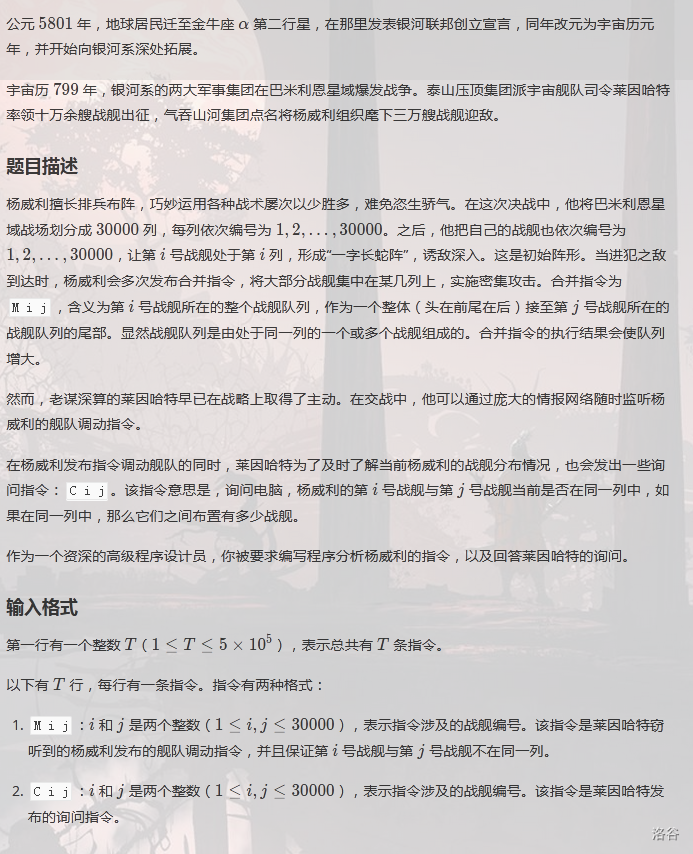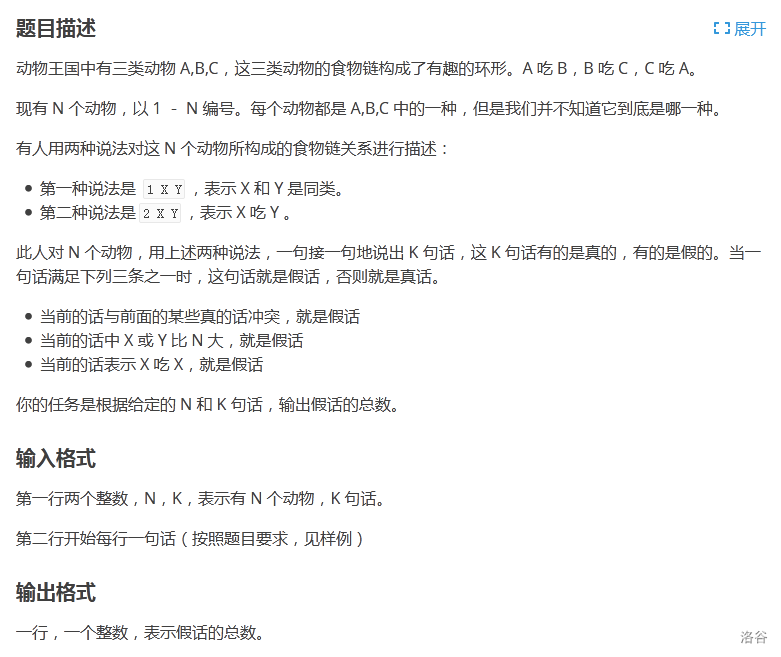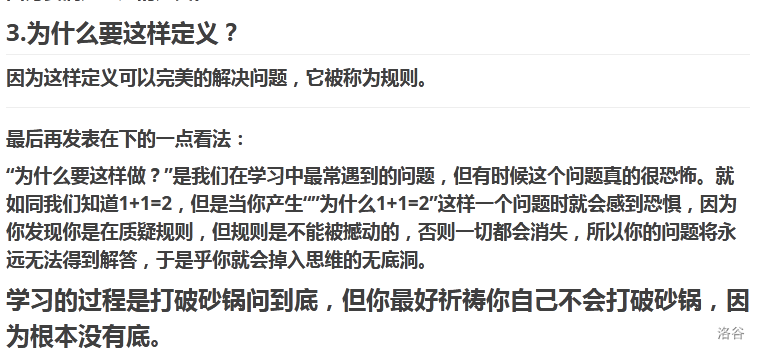# 介绍

## 1.带权并查集部分

• $X$$Y$ 之间差了几代，如果不在一个家族里就输出 $-1$

P1196 银河英雄传说f[i]表示i的祖先

front[i]表示i到祖宗的距离(即隔了几代)

num[i]表示以i为队头家族的长度



		f[fa]=fb;
front[fa]+=num[fb];
num[fb]+=num[fa];
num[fa]=0;


inline int find(int x)
{
if(f[x]==x) return f[x];
int fx=find(f[x]);
front[x]=front[x]+front[front[fx]];//仔细想想这行有啥用
return fx;
}


## 完整Code

#include<cstdio>
#include<iostream>
#include<cmath>
using namespace std;
int t;
char inm;
int a,b;
int f;//i的祖先
int front;//表示i到目前队头的距离
int num;//num[i]表示以i为队头的队列长度
inline int find(int x)
{
if(f[x]==x) return f[x];
int fx=find(f[x]);
front[x]+=front[f[x]];
return f[x]=fx;
}
int main()
{
cin>>t;
for(int i=1; i<=30005;i++)
{
f[i]=i;
front[i]=0;
num[i]=1;
}
while(t--)
{
cin>>inm>>a>>b;
int fa=find(a);
int fb=find(b);
if(inm=='M')
{
f[fa]=fb;
front[fa]+=num[fb];
num[fb]+=num[fa];
num[fa]=0;
}
if(inm=='C')
{
if(fa==fb)
{
cout<<abs(front[a]-front[b])-1<<endl;
}
else
{
cout<<-1<<endl;
}
}
}
return 0;
}


## 2.种类并查集部分

P2024 [NOI2001] 食物链A 吃 B，B 吃 C，C 吃 A，我们整理一下，用“天敌”和“同类”“猎物”来表示关系，不难发现他们之间的关系

（同类表示同一种动物，天敌表示谁吃谁，猎物表示谁被谁吃）

1. 若X为Y的同类，他们所有的天敌和猎物就会在一个集合里

2. 若X为Y的天敌，Y的天敌和X就会在一个集合里，Y的猎物和X的天敌也是（画个图试试），X的猎物和Y也是

• 1.$1-n$ 的范围存储这个动物的同类

• 2.$n+1-2n$ 的范围存储这个动物的天敌

• 3.$2n+1-3n$ 的范围存储这个动物的猎物

### First 当X与Y是同类时

• 1.$x>n$ 或者 $y>n$

• 2.$x+n$$y$ 在同一个集合（天敌关系）

• 3.$x+2n$$y$ 在同一个集合（猎物关系）

### Second 当X与Y是吃和被吃关系时

• 1.同上

• 2.$x$$y$ 在同一个集合（同种不可能互相残杀）

• 3.$x$$y$ 相等（自己吃自己？）

• 4.$x+n$$y$ 在同一个集合（反了）

join(x,y);
join(x+n,y+n);
join(x+2*n,y+2*n);


join(x+2*n,y);//x的猎物和y合并(天敌的猎物就是同类)
join(x,y+n);//x和y的原有天敌合并
join(x+n,y+2*n);//x的天敌和y的猎物合并


#include<cstdio>
#include<iostream>
using namespace std;
int f;
int n,k,ans;
int a,x,y;
inline int find(int xx)
{
if(f[xx]==xx)
{
return f[xx];
}
return f[xx]=find(f[xx]);
}
inline void join(int xx,int yy)
{
int f1=find(xx),f2=find(yy);
if(f1!=f2) f[f1]=f2;
return;
}
int main()
{
cin>>n>>k;
for(register int i=1; i<=3*n;i++) f[i]=i;
while(k--)
{
scanf("%d%d%d",&a,&x,&y);
if(a==1)//x和y是同类
{
if(x>n||y>n||find(x+n)==find(y)||find(x+2*n)==find(y)) ans++;//1.x，y大于n 2.y是x天敌 3.y是x猎物
else
{
join(x,y);
join(x+n,y+n);
join(x+2*n,y+2*n);//所有的都是相同的
}
}
if(a==2)
{
if(x==y||x>n||y>n||find(x)==find(y)||find(x+n)==find(y))//1.同上 2.x和y相等（自己吃自己） 3.x和y是同类 4.y是x的天敌
{
ans++;
}
else
{
join(x+2*n,y);//x的猎物和y合并(天敌的猎物就是同类)
join(x,y+n);//x和y的原有天敌合并
join(x+n,y+2*n);//x的天敌和y的猎物合并
}
}
}
cout<<ans;
}


## 结束语posted @ 2021-07-03 20:05  Edolon  阅读(36)  评论(0编辑  收藏  举报
Live2D